# 唯一路径的动态规划解法，阿里巴巴架构演化路径 John 易筋 ARTS 打卡 Week 07John(易筋)

# 1. Algorithm: 每周至少做一个 LeetCode 的算法题

62. Unique Paths

A robot is located at the top-left corner of a m x n grid (marked 'Start' in the diagram below).

﻿

The robot can only move either down or right at any point in time. The robot is trying to reach the bottom-right corner of the grid (marked 'Finish' in the diagram below).

﻿

How many possible unique paths are there?

﻿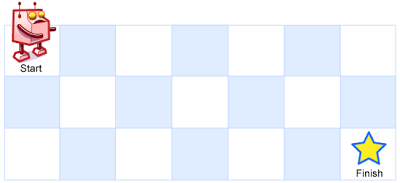﻿

Above is a 7 x 3 grid. How many possible unique paths are there?

﻿

Example 1:

Input: m = 3, n = 2Output: 3Explanation:From the top-left corner, there are a total of 3 ways to reach the bottom-right corner:1. Right -> Right -> Down2. Right -> Down -> Right3. Down -> Right -> Right

﻿

Example 2:

Input: m = 7, n = 3Output: 28

﻿

Constraints:

﻿

• 1 <= m, n <= 100

• It's guaranteed that the answer will be less than or equal to 2 * 10 ^ 9.

﻿

## 1 动态规划解法

1. 往下走的列path[i]都初始化为1，表示一直往下有一种的解法；往右走的行path[j]都初始化为1，表示一直往右有一种的解法。

2. 再看第二列，当前位置的记录是由上面的解法，和左边的解法，加起来就是当前的解法。所以 2 = 1 + 13 = 2 + 1. 以此类推。

3. 结果是path[m-1][n-1], 因为是从0开始计算的。

﻿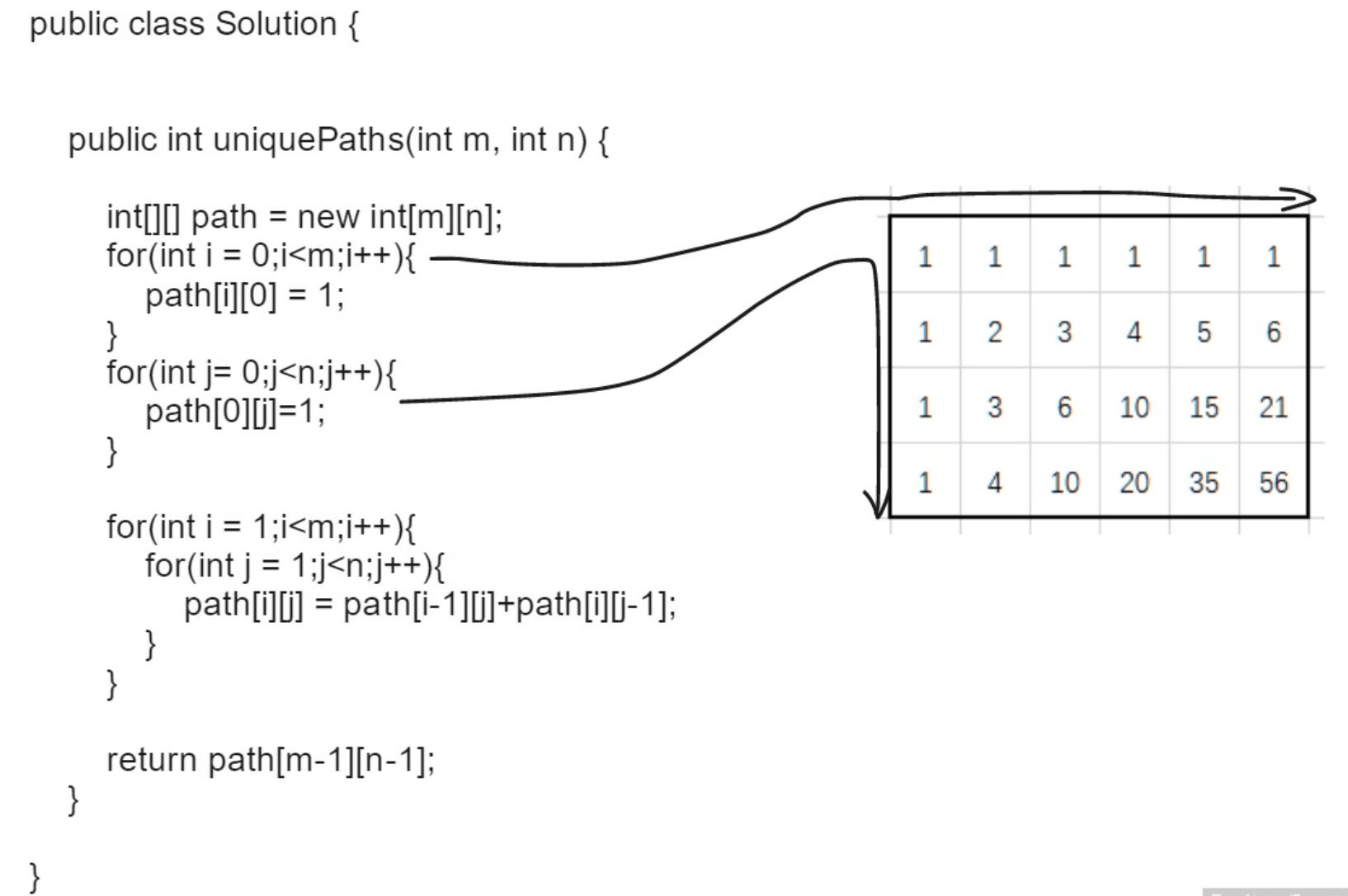﻿

public int uniquePaths(int m, int n) {    int[][] dp = new int[m][n];    for (int i = 0 ; i < m; i++) {      dp[i] = 1;    }    for (int k = 0; k < n; k++) {      dp[k] = 1;    }    for (int i = 1; i < m; i++) {      for (int k = 1; k < n; k++) {        dp[i][k] = dp[i - 1][k] + dp[i][k - 1];      }    }﻿    return dp[m - 1][n - 1];  }

﻿

## 2 二项系数解法Binomial coefficient

﻿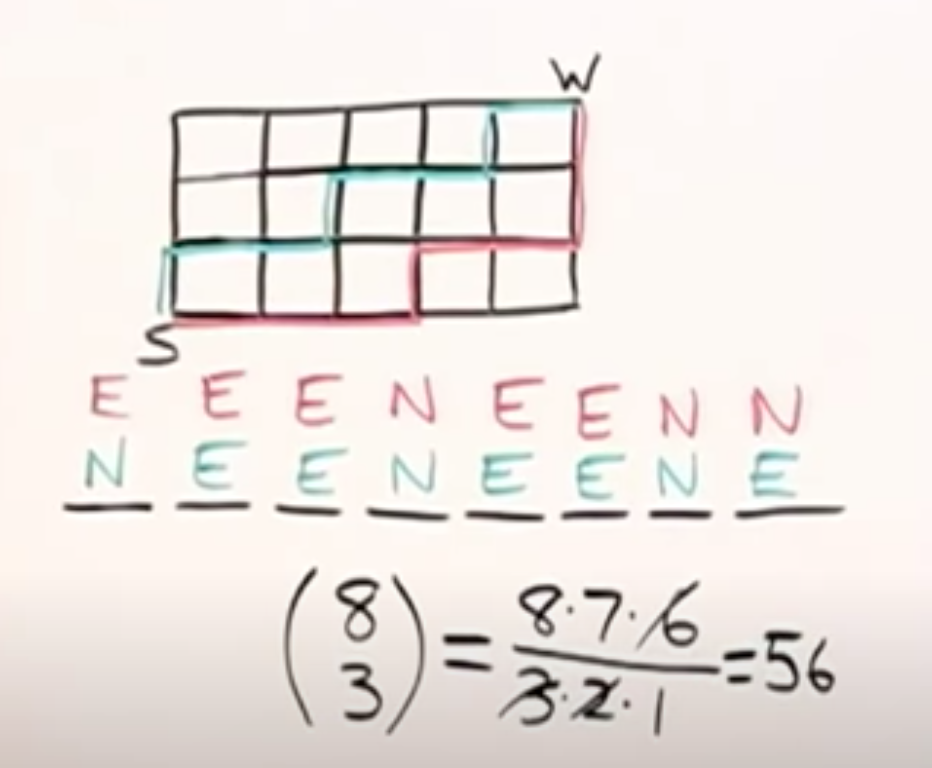﻿

class Solution {    public int uniquePaths(int m, int n) {        int totalStep = m + n - 2;        int change = (m > n ? n : m) - 1;        double count = 1;        for (int i = 1; i <= change; i++) {          count = count * totalStep / i;          totalStep--;        }        return (int)count;    }}

﻿

﻿

# Top 10 Trending iOS Projects at the Start of 2020

https://medium.com/better-programming/top-10-trending-ios-projects-at-the-start-of-2020-62dfff1707e0

﻿

# 3. Tips: 学习至少一个技术技巧

## 博客：

﻿﻿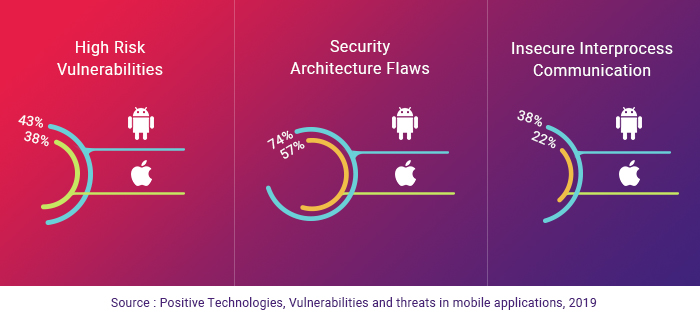﻿

# 什么是RASP？﻿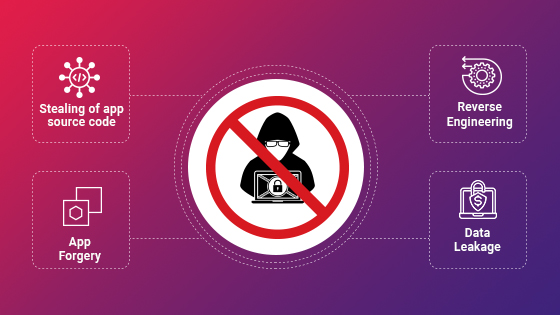﻿

# 4. Share: 分享一篇有观点和思考的技术文章

﻿

﻿

﻿

﻿

20年前的系统架构主要是面对大型企业：IBM、SUN、Microsoft、Oracle、沃尔玛、家乐福、用友、金蝶、联想等。

﻿

Google 日均 PV 数 35 亿，日均 IP 访问数 3 亿。

﻿

﻿

﻿

﻿

﻿

﻿

﻿

﻿

﻿

# 参考

https://leetcode.com/problems/unique-paths/

﻿## 评论